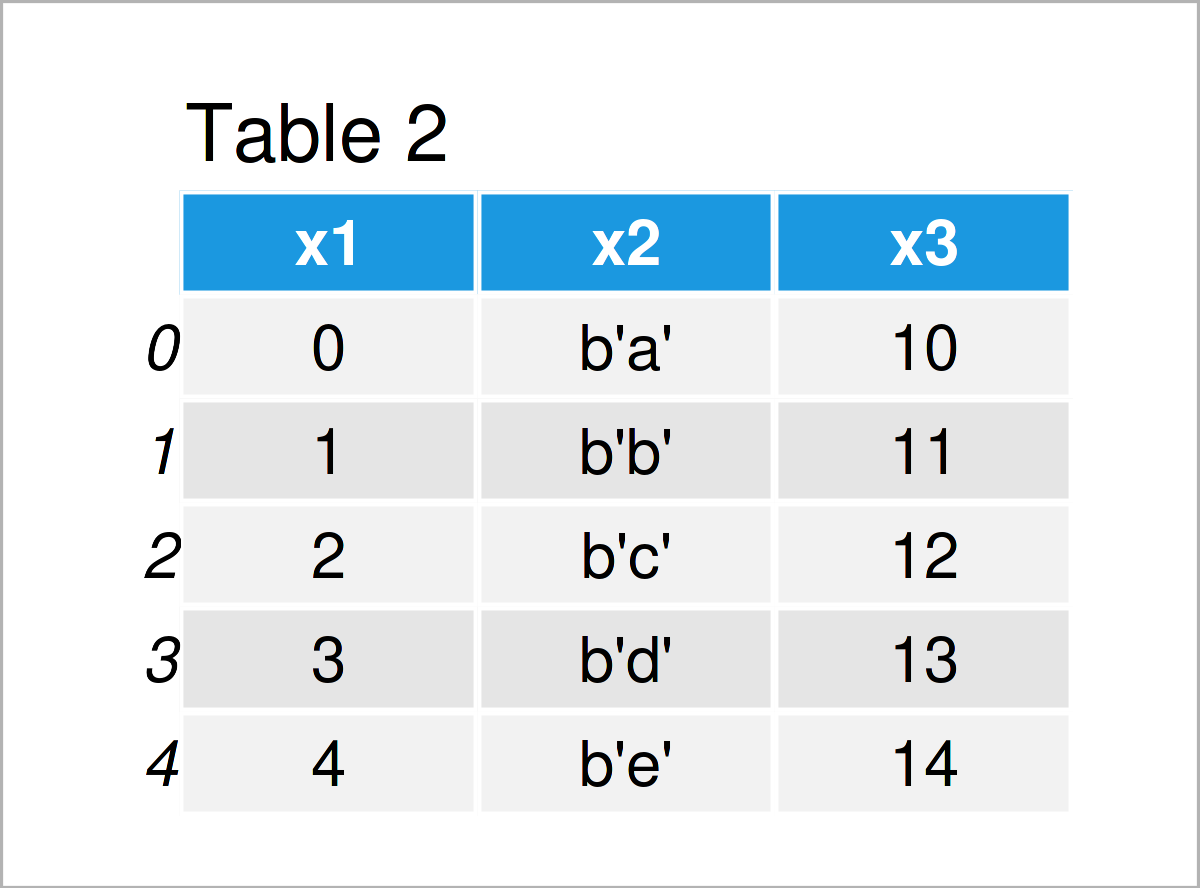# Convert Object Data Type to String in pandas DataFrame Column in Python (2 Examples)

In this Python post you’ll learn how to convert the object data type to a string in a pandas DataFrame column.

The page will consist of these contents:

Let’s dive right into the tutorial!

## Example Data & Add-On Libraries

We first have to load the pandas library to Python:

`import pandas as pd                       # Load pandas`

We’ll also have to construct some data that we can use in the examples below:

```data = pd.DataFrame({'x1':range(0, 5),    # Create pandas DataFrame
'x2':['a', 'b', 'c', 'd', 'e'],
'x3':range(10, 15)})
print(data)                               # Print pandas DataFrame```Have a look at the previous table. It shows that our example data consists of five rows and three columns.

Let’s check the data types of the columns in our pandas DataFrame:

```print(data.dtypes)                        # Print data types of columns
# x1     int64
# x2    object
# x3     int64
# dtype: object```

As you can see, the columns x1 and x3 are integers, and the column x2 has the object data type.

This might be surprising, since the column x2 obviously contains character strings.

In the following examples, I’ll explain why this is the case. So keep on reading!

## Example 1: astype() Function does not Change Data Type to String

In case we want to change the data type of a pandas DataFrame column, we would usually use the astype function as shown below:

`data['x2']= data['x2'].astype(str)        # Applying astype function`

However, after running the previous Python code, the data types of our columns have not been changed:

```print(data.dtypes)                        # Print data types of columns
# x1     int64
# x2    object
# x3     int64
# dtype: object```

The reason for this is that data types have a variable length. Hence, strings are by default stored as the object data type.

In other words: If a pandas DataFrame column has the object dtype, you can usually consider it as a string.

However, there’s one little workaround that I want to show you in the next example.

## Example 2: Define String with Manual Length in astype() Function

In Example 1, I have explained that data types have a variable length, and for that reason, strings are automatically set to the object dtype.

There is usually no reason why you would have to change that data type. However, in this example, I’ll show how to specify the length of a string column manually to force it to be converted to the string class.

To accomplish this, we can specify ‘|S’ within the astype function as shown below. This sets the string length to the maximum string lengths in our DataFrame column (i.e. 1):

```data['x2']= data['x2'].astype('|S')       # Applying astype function
print(data)                               # Print updated pandas DataFrame```In Table 2 you can see that we have created an updated version of our pandas DataFrame using the previous Python programming code.

In this new DataFrame, you can see a b in front of the values in the column x2. The b stands for bytes, and you can learn more about this here.

However, let’s check the dtypes of our updated DataFrame columns:

```print(data.dtypes)                        # Print data types of columns
# x1    int64
# x2      |S1
# x3    int64
# dtype: object```

The column x2 has been converted to the |S1 class (which stands for strings with a length of 1).

## Video, Further Resources & Summary

I have recently published a video on my YouTube channel, which illustrates the Python programming syntax of this article. You can find the video below:

In addition to the video, you might read the other tutorials on this homepage. You can find some related tutorials below:

Summary: You have learned in this tutorial how to transform the object data type to a string in a pandas DataFrame column in the Python programming language. Please let me know in the comments, in case you have additional questions.

Subscribe to the Statistics Globe Newsletter